Courses

# Polyphase Synchronous Machines - 2 Notes | EduRev

## Electrical Engineering (EE) : Polyphase Synchronous Machines - 2 Notes | EduRev

The document Polyphase Synchronous Machines - 2 Notes | EduRev is a part of the Electrical Engineering (EE) Course Electrical Engineering SSC JE (Technical).
All you need of Electrical Engineering (EE) at this link: Electrical Engineering (EE)

SYNCHRONOUS MOTOR PHASOR DIAGRAM
• The voltage equation for a synchronous motor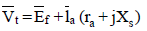Remember:-
• For an alternator, phasor Ef is always ahead of phasor Vt , just as field poles are ahead of ør , For a synchronous motor, phasor Ef is always behind phasor vt , just as the filld poles are behind ør,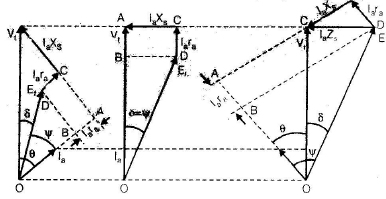For lagging power factor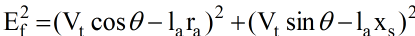For unity power factor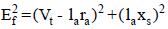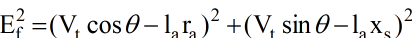POWER FLOW THROUGH AN IMPEDANCE
• Two ac voltage source E1 and E2 interconnected through an impedance Z∠θZ. With the current l flowing from E1 to E2.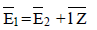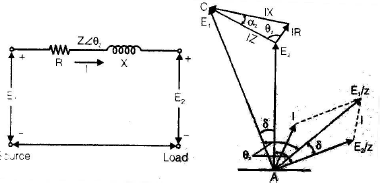or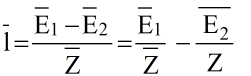Here impedance angle θis given by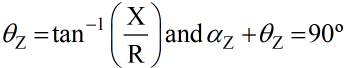ACTIVE POWER
• The power at the source end of the impedance P1 = E1 (component of l in phase with E1)

or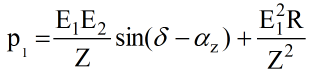• The power at the load end P2 = E2 (Component of in phase with E2)

or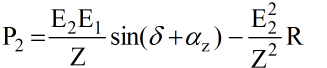Power Flow in Cylindrical Rotor Synchronous Machine.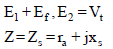• Power input to generator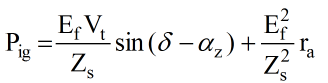• Power output of generator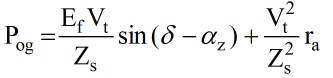For a cylindrical rotor synchronous motor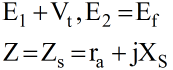• Power input to motor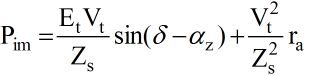• Power output of motor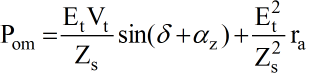NOTE:-
• The power at the shaft for a synchronous motor is Pom minus the rotational losses (friction, windage and core losses). Here Pom is called the mechanical power developed or gross power developed. Similarly the mechanical power input to generator is Pg plus the rotational losses.

Remember:
• The difference between input (pig or pim) and output (Pog or pom), for either a generator or a motor, must be equal to ohmic loss  la2 ra .

• Generally, armature resistance ra is negligible

az = 0  and Zs = Xs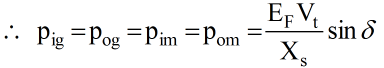• Power input to generator is at the terminals where the voltage is Ef and power output of the motor Pom is also at the same terminals. The powers Pig and Pom Should therefore be equal and opposite to each other but with δ replaced by (-δ)

Pom = Pig

Similarly Pim = - Pog

maximum Power Conditions
For maximum power output
load angle δ = impedance angle θz.
• maximum power output from a generator is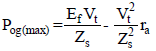• maximum power out ( or maximum power developed) in case of a motor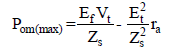For maximum power input
Load angle , δ = 90 + αZ = 180 - impedance angle θz.
• Maximum power input to generator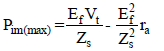• Maximum power input to motor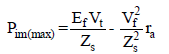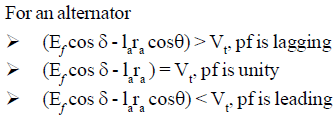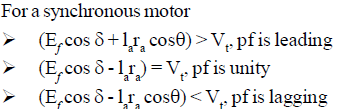Reactive Power
• Reactive power at the generator output terminals Qog = E2 (component of l in quadrature lagging E2)

or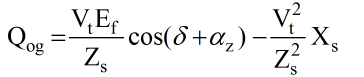• Output terminal for the generator are same as the input terminals for the motor.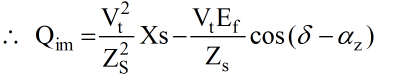Condition For maximum Reactive Power
For a generator

δ + αz = 0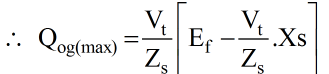or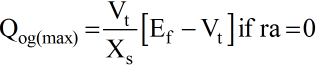For a motor

δ - αz = 0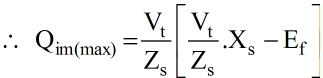or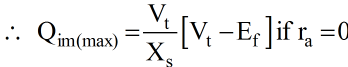when Pim is maximum, δ = 90 + αz , the reactive power under this condition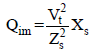Generating Mode
If ra = 0 then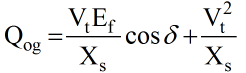or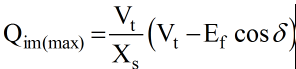Remember:-

• For a motor, positive values of active and reactive powers indicate their flow into the motor. Fdifferent excitations, the flow of reactive power in a motor,
• When Ecosδ > V(overexcited), Qim is negative and therefore reactive power flow is outward, i.e, motor is acting as a generator of reactive power . In other words, the motor is delivering reactive power to the infinite bus and is operating at a leading pf.
• When Ecosδ > Vt (normally excited), Qog = 0. generator neither delivers nor absorbs reactive power. Under this condition, synchronous generator pf is unity.
• When Ecosδ > Vt (under excited), Qim is positive and reactive power flow is inward, i.e, motor is absorbing reactive power just as it is absorbing active power. Under this condition, synchronous motor pf is lagging.

Remember:

• Under the condition of maximum reactive power input, the reactive power Q im (max) is positive, the synchronous motor is therefore absorbing reactive power.
• Under the condition of maximum active power input, reactive power Qim is positive. This means that synchronous motor absorbs reactive power under this condition also.
• Under the conditions of maximum values of active or reactive power, a synchronous machine always absorbs reactive power.

NOTE:
• An overexcited synchronous machine delivers reactive power whereas an underexcited one absorbs reactive power. Under normal excitation, it neither absorbs nor delivers reactive power.

POWER FACTOR CONTROL
• Power factor of synchronous machine is control by the adjustment of their field excitation.

Synchronous Motor

• AC electromagnetic device must draw a magnetizing current a.c. source to establish the working flux. This magnetizing current lags the applied voltage by almost 90º. Magnetizing current or lagging VA drawn by a.c. electromagnetic device is to set up the flux in the magnetic circuit of the device.
• Armature winding is excited by a.c. source and field winding by d source when motor is working at constant applied voltage, the resultant air gap flux as demanded by constant Vt remains constant.
• This resultant air gap flux is established by the cooperation of both ac in armature winding and dc in field winding.
• If the field current is sufficient enough to set up the air gap flux demanded by constant Vt, then magnetizing current or lagging VA required from the ac source is zero and therefore, the motor operates at unity power factor.
• This field current causes unity power factor operation of synchronous motor and is called normal excitation or normal field current.
• If field current is made less than the normal excitation i.e. the motor is under excited then the deficiency in flux must be made by the armature winding m.m.f. The armature winding draw a magnetizing current or lagging reactive VA from the a.c. source and as a result of it motor operates at a lagging power factor.
• If field current is made more than its normal excitation i.e. the motor is overexcited than the excess flux must be neutralized by the armature winding.
• per phase power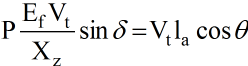• For constant power output, Ef sinδ and la cosθ must remain constant because Vt and Xs are constant.
• As the field current is varied excitation voltage Ef varies but component of Ef normal to Vt. i.e. Ef sinδ must remian constant.
• As Ef varies, laXs and therefore armature current la also varies but in such a manner to keep la cosθ constant.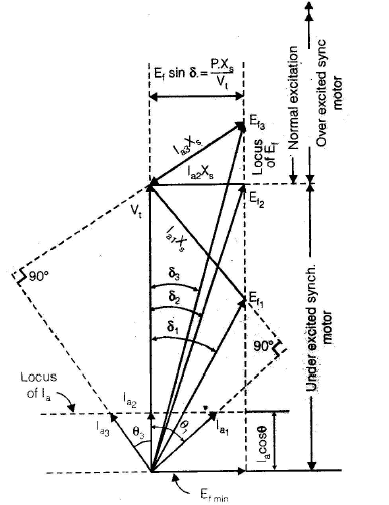Effect of field current on synchronous motor power factor

• When the excitation voltage is  Ef1 , the motor is underexcited and the armature current lf1 must lag Vt by power factor angle θ1 so that relation (E+ jla Xs = Vt) is satisfied.
• When excitation is increased to Ef2 by increasing the field current the angle must decrease from δto δ2 so that Ef1 sin δ1 = Ef1 sin δ2. For satisfying relation (Ef + jlaXs =Vt) armature current must change Ia2
• When angle δ2 decreases to δ3 so that ( Ef1 sin δ1 = Ef1 sin δ= Ef3 sin δ3) current Ia3 must change and motor is working at leading power factor and the active power component of armature current are equal ( Ia1cos θ1 = Ia2 cos θ2 = Ia2 cos θ3) and current at unity power factor is minimum and more at leading and lagging power factor.
• The variation of armature current with the change in field curve
• The unity pf dotted curve joining all the minimum armature current points, is called unity power factor compounding curve similarly compounding curve for 0.8 p.f. lag and 0.8 p.f. Lead.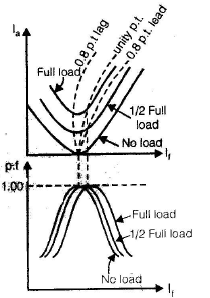Synchronous motor V-curves and power factor versus field current curves

• If motor power factor is plotted as a function of the field current the curve as shown above is obtained.
• Field current for u.p.f. at full load is more than the field current for u.p.f at No load.
• If the synchronous full load is operating at upf, then removal of the shaft load causes the motor to operate at a leading pf.
• The curve obtained by plotting power factor versus field current resemble inverted V, called inverted V-carves of synchronous motor.
• The ability of a synchronous motor to operate at leading power factors when overexcited, is utilized for improving the power factor.
• When the primary function of a synchronous motor is to improve the power factor of the system or to control the flow of kVAr, then the synchronous motor carries no mechanical load on its shaft. Such an unloaded synchronous motor with no shaft extension, is called a synchronous condenser or synchronous compensator and is used in large power systems, where static capacitors are uneconomical.
• As la leads Vt by 90º just like a capacitor, synchronous motor behaves like a capacitor bank under zpf leading operation.
• As la lags Vby 90º just like an inductor synchronous motor behaves like an inductor bank under zpf lagging operation.
• The V-curve of an ideal synchronous motor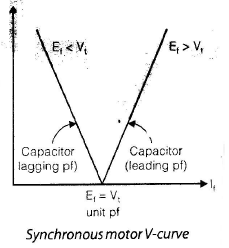Alternator
• When excitation emf is Ef1, the alternator is underexcited and the armature current la1 is leading V= Vb = bus-bar voltage.
• For Ef1 , underexcited alternator operates at a leading and absorbs reactive power from infinite bus.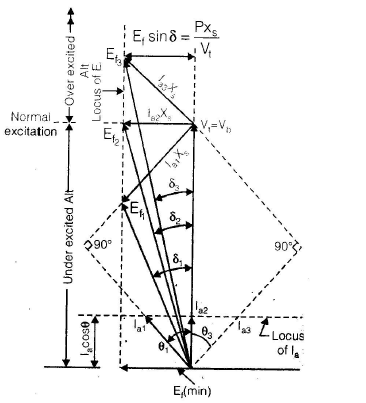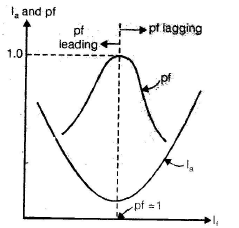Effect of field current on an alternator connected to infinite bus

• With an increase in field current, excitation emf rises. For excitation emf Ef2 , armature current la2 is im phase with Vt = Vb. Under unity pf operation, the alternator is said to be normally excited and it neither delivers nor absorbs reactive power. For Ef3 more than Ef2, la3 lag Vt. The overexcited alternator operates at a lagging pf and delivers reactive power to infinite bus.
• Just like a synchronous motor, plot of la versus lis called V-curve of a alternator connected to an infinite bus. The power factor versus field current curve, known as inverted V-curve.

POWER-ANGEL CHARACTERISTICS
Cylindrical-rotor synchronous Machine

• A generator and is feeding power to an infinite bus of constant voltage Vt.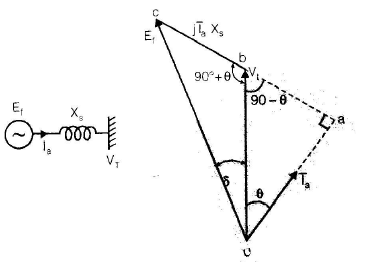Salient pole synchronous generator single line diagram and phasor diagram for a lagging pf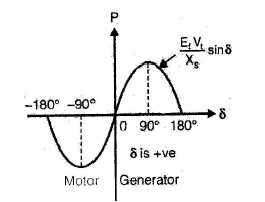• The per phase power delivered to the infinite bus

P=Vtla cosθ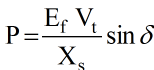Salient-pole Synchronous Machine
• The per phase power delivered to the bus.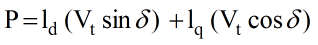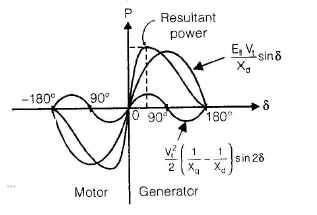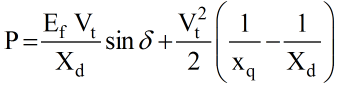• The total power consists of a fundamental component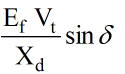and a second harmonic component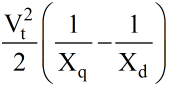sin2δ

• For a salient pole synchronous generator, the per phase reactive power in terms of power angle δ and for a lagging power facor is given by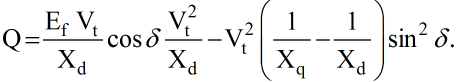• The synchronizing power coefficient is a measure of the stiffness of electromagnetic coupling between stator and rotor fields. Too large stiffness of coupling means that the motor tends to follow closely, the variation of speed caused by the disturbance in electric power supply. In case there is no power-supply disturbance, then too much stiffness coupling would cause the motor speed to remain practically constant, regardless of the mechanical load fluctuations.

SYNCHRONOUS MACHINE STABILIYT
• A synchronous machine connected to an infinite bus is said to be working in a stable condition, if it is in synchronism or in step with the bus.

Stability
• The tendency of a synchronous mechine to develop forces so as to maintain synchronism and equilibrium is called stability.

Stability Limit
• A stability limit represents the maximum power flow possible, when the synchronous machine is operating with stability.

• The maximum power flow possible through a particular point without loss of stability, when the power is increased very gradually.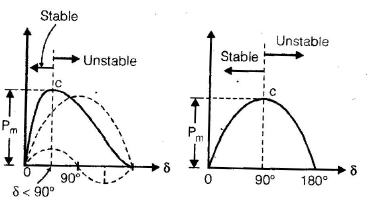• The steady-state limit can be improved upon as follows:

1. Synchronous machine excitation should be increased, so that Ef becomes more.
2. Reactance should be decreased, e.g. two transmission lines in parallel would reduce the line reactance to half.
3. Series capacitors may be used to reduce the line reactance.

HUNTING
• A synchronous machine operates satisfactorily, if the mechanical speed to the rotor is equal to the stator field speed.
• Any departure from these conditions, gives rise to synchronizing forces which tend to maintain this equality.
• Phenomenon, involving the oscillations of the rotor about its final equilibrium position, is called hunting.
• During the rotor oscillations or hunting. the orientation of phasor Ef changes relative to fixed voltage Vt and because of this reason, hunting is also called phase-swinging.

Reduction of Hunting
• Damper windings.
• Use of flywheels which helps in maintaining the
rotor speed constant.
• By using suitable synchronizing power coefficient.

DAMPER WINDINGS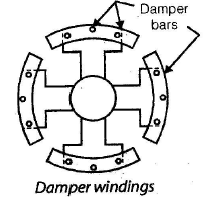• Damper windings consist of low- resistance copper. brass or aluminium bars embedded in slots in the pole-faces of salient-poles machines. The projecting ends of the bars are connected  to short circuiting strips of the same material asused for the bars. Sometimes interpolar connectors are omitted to form incomplete type of damper winding.

NOTE:
• In damper winding techniques, for reducing hunting resistance (Rd) of damper windings should be minimum, While for good starting torque, damper bars should have a low resistance therefore we have to make compromise in the value of damper winding resistance. it should be in between two.
• In alternator, the value of damper winding resistance should be low for minimizing hunting.

EFFICIENCY OF SYNCHRONOUS MACHINES
• The various losses in synchronous machines are:

(a) friction and winding loss and
(b) open circuit core loss.

• Field circuit loss

(c) l2 R loss in armature winding.

(d) lR loss in armature winding.

(e) in iron and
(f) in the armature conductors.

• The combination of direct load loss and stray load losses is referred to as shot circuit load loss.

The Stray load loss consists of two components, namely:
• iron losss or core loss due to armature leakage flux and
• armature ohmic loss due to skin effect and eddy currents in the armature conductors.

POWER FLOW DIAGRAMS

Pr = Friction and windage loss + open-circuit loss pr + vf l= constant losses Short=circuit load loss -  3la2.ra + stray- load loss.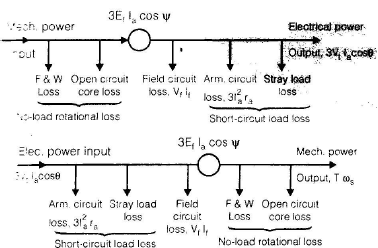• Maximum efficiency in a synchronous machine occurs when variable losses = constant losses. or 3la2.ra = P+Vf .lwhere lam is the armature current at which maximum efficiency occurs in the synchronous machine.
• Total alternator losses = F.W.loss + open-circuit core loss + short-circuit load loss + field - circuit loss.

= W+ W2 + W3 + Vf lf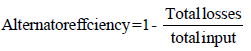STARTING OF SYNCHRONOUS MOTOR
• A synchronous motor is not self starting. It can be started by the following two methods.

• Startin with the help of an external prime mover.
• Startin with the help of damper windings.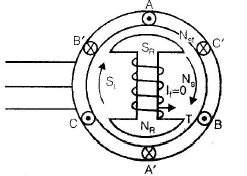Stator field rotates at NS
at starting Nr = 0
Let, Ns = 1500 rpm

1 rev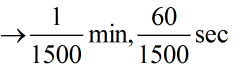half rev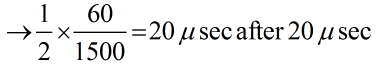At starting rotor field stationary and stator field is rotating at h higher speed that is synchronous. Due to the interaction between rotor and stator poles, the torque developed reverses after each half revolution of stator field. Due to its inertia rotor can not respond so quickly hence it remains stationary that's whey synchronous motor is non self-stationary that's why synchronous motor is non self- starting if by some external means rotor is made to rotate at a speed close to synchronous then due to the locking of stator and rotor field rotor will continue to rotate at synchronous speed.

Damper winding
• Solid copper (cu) or Aluminium (Al) are embedded in the slots cut in the pole shoe and short circuited by end rings like squirrel cage induction motor (SCIM).
At starting no-field excitation is given, so there is relative motion between damper winding and stator field.
Ns - Nr = Ns - 0 = Ns
• AT Starting no excitation is given to the rotor, Due to the relative motion between damper winding and stator field the induced current in damper winding opposes the relative motion and hence starting torque is produced due to induction motor principle and rotor start rotating at a speed some what less than synchronous. Now dc excitation is given and stator and rotor field remains continue to rotates at synchronous speed, when rotates at synchronous speed there is no relative motion between damper winding and stator field. No induced current in the damper winding hence no role of the damper winding in steady state.

Offer running on EduRev: Apply code STAYHOME200 to get INR 200 off on our premium plan EduRev Infinity!

65 docs|37 tests

,

,

,

,

,

,

,

,

,

,

,

,

,

,

,

,

,

,

,

,

,

;